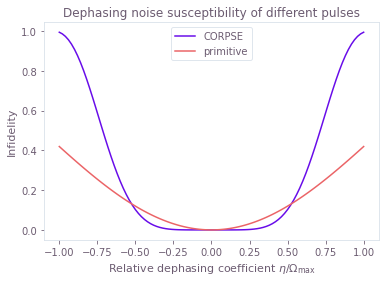# Calculate quasi-static error susceptibility

Characterizing the robustness of a pulse to quasi-static noise

The Q-CTRL Python package enables you to evaluate the susceptibility of quantum controls to quasi-static noise on multiple simultaneous noise channels. This process can provide a useful characterization of the robustness of candidate controls. In this notebook we show how to compute quasi-static scans using the Q-CTRL Python package.

## Imports and initialization

All usage of the Q-CTRL Python package begins by importing the qctrl package and starting a session.

import matplotlib.pyplot as plt
import numpy as np
from qctrlvisualizer import get_qctrl_style

plt.style.use(get_qctrl_style())

# Predefined pulse imports
import qctrlopencontrols

from qctrl import Qctrl

# Starting a session with the API
qctrl = Qctrl()


## Worked example: Susceptibility to simultaneous amplitude and dephasing noise

In this example we will compare a series of composite $\pi$ pulses applied to a single qubit under amplitude and dephasing noise. The Hamiltonian of the quantum system is:

\begin{align*} H(t) = &\frac{1+\beta(t)}{2}\Big( \Omega(t) \sigma_- + \Omega^*(t) \sigma_+ \Big) + \frac{\eta(t)}{2} \sigma_z \end{align*}

where $\Omega(t)$ is a time-dependent Rabi rate, $\beta(t)$ is a fractional time-dependent amplitude fluctuation process, $\eta(t)$ is a small slowly-varying stochastic dephasing noise process, $\sigma_\pm = (\sigma_x \mp i \sigma_y)/2$, and $\sigma_k$ are the Pauli matrices.

In a 2D quasi-static scan, we scan across a two-dimensional grid of values $(\beta,\eta)$ for $\beta(t)\equiv \beta$ and $\eta(t)\equiv\eta$, and calculate the infidelity of the resulting gate in each case. Comparing the variation in infidelity across the grid gives information about the robustness of the appropriate control to simultaneous quasi-static noise on the two channels.

### Control schemes

We consider the following driven control schemes for the controllable $\Omega(t)$ term, which are available from Q-CTRL Open Controls and described in the reference documentation: primitive, BB1, SK1, and CORPSE. In the following cell, we create a list of dictionaries containing the Q-CTRL Open Controls functions we will simulate.

We also define the different parameters in the system, such as the total Rabi rotation we want to achieve ($\pi$), the maximum Rabi rate allowed ($\Omega_{\mathrm{max}}$), and the arrays defining the grid of noise coefficients in the quasi-static scan. In particular, we use a grid with 51 points for $\beta\in [-0.5,0.5]$ and 101 points for $\eta\in[-\Omega_{\mathrm{max}}, \Omega_{\mathrm{max}}]$.

# Define standard matrices
identity = np.array([[1, 0], [0, 1]], dtype=complex)
sigma_x = np.array([[0, 1], [1, 0]], dtype=complex)
sigma_z = np.array([[1, 0], [0, -1]], dtype=complex)
sigma_m = np.array([[0, 1], [0, 0]], dtype=complex)

# Define schemes for driven controls to compare
schemes = {
name: {"function": function}
for name, function in [
("primitive", qctrlopencontrols.new_primitive_control),
("BB1", qctrlopencontrols.new_bb1_control),
("SK1", qctrlopencontrols.new_sk1_control),
("CORPSE", qctrlopencontrols.new_corpse_control),
]
}

# Define control parameters
total_rotation = np.pi
omega_max = 2 * np.pi * 1e6  # Hz

# Define coefficient arrays for the noise values
amplitude_coefficients = np.linspace(-0.5, 0.5, 51)
dephasing_coefficients = np.linspace(-1.0, 1.0, 101) * omega_max


### Creating the graph

As described in the Setting up quantum systems user guide, we will build a graph that we will execute with the qctrl.functions.calculate_graph function to perform the computation. For convenience, we wrap this calculation in a function that takes a pulse given by a Q-CTRL Open Controls function, defining the piecewise-constant (PWC) pulses for $\Omega(t)$. The function returns the infidelities for each pair of values of the noise coefficients $\beta$ and $\eta$.

In order to perform the quasi-static scan, we parallelize the calculation of the infidelity for all possible values of the dephasing and amplitude noises by creating a two-dimensional batch of Hamiltonians, with each element of the batch corresponding to a pair of amplitude and dephasing coefficient values.

To achieve that, we use functions in the qctrl.operations namespace to define PWC signals for each term:

• the time-dependent Rabi signal as a [51, 1] batch (for each value of $\beta$),
• the constant dephasing term as a [1, 101] batch (for each value of $\eta$).

We can then multiply each of these by their corresponding operator to create the corresponding Hamiltonian term, and add them together to obtain the total Hamiltonian. When the terms are added, as the batches for both quasi-static noises correspond to different dimensions, we obtain a [51, 101] batch of 2×2 PWC Hamiltonians (when adding PWCs, their value and batch dimensions are broadcasted separately). As a result, the infidelities returned by the qctrl.operations.infidelity_pwc node are a [51, 101] tensor, where each element gives the infidelity associated with a pair of amplitude and dephasing coefficient values.

With the graph built, we calculate the quasi-static scans by calling the qctrl.functions.calculate_graph function (with the graph we defined and the output_node_names we want to obtain). Finally, we extract the infidelities from result.output["infidelities"]["value"] (where result is the object returned by qctrl.functions.calculate_graph).

def calculate_quasi_static_scan(pulse):

# Start graph definition
with qctrl.create_graph() as graph:

# [51, 1, T] array with values of (1+β)Ω(t) on each segment and for each value of β
complex_rabi_rates_with_noise = (
(1 + amplitude_coefficients[:, None, None])
* pulse.rabi_rates
* np.exp(1j * pulse.azimuthal_angles)
)
# [51, 1] batch of PWC signals for (1+β)Ω(t)
# We specify time_dimension=2, as the PWC values array has two batching dimensions.
rabi_signal = qctrl.operations.pwc(
values=complex_rabi_rates_with_noise,
durations=pulse.durations,
time_dimension=2,
)
# [51, 1] batch of 2×2 PWC operators for the coupling term, (1+β)[Ω(t)σ- + Ω*(t)σ+]
rabi_coupling_term = qctrl.operations.pwc_operator_hermitian_part(
rabi_signal * sigma_m
)

# [1, 101] batch of 2×2 constant (with a single segment) PWC signals for η
dephasing_signal = qctrl.operations.pwc(
values=dephasing_coefficients[None, :, None],
durations=np.array([pulse.duration]),
time_dimension=2,
)
# [1, 101] batch of 2×2 PWC operators for the dephasing drift term, η σz/2
dephasing_term = dephasing_signal * sigma_z / 2

# [51, 101] batch of 2×2 PWC operators for the total Hamiltonian,
# with each element in the batch represents a unique pair of (β, η) values
# (the [51, 1] and [1, 101] batches get broadcasted to [51, 101]).
hamiltonian = rabi_coupling_term + dephasing_term

# [51, 101] tensor with the infidelities
qctrl.operations.infidelity_pwc(
hamiltonian=hamiltonian,
target_operator=qctrl.operations.target(sigma_x),
name="infidelities",
)

# Execute the graph
result = qctrl.functions.calculate_graph(
graph=graph, output_node_names=["infidelities"]
)

# Extract and return infidelities
return result.output["infidelities"]["value"]


### Executing the graph and extracting the infidelities

We can now call the function for each Q-CTRL Open Controls scheme and extract the calculated infidelities. We also store the values for the noise coefficients in the quasi-static scan to plot them later.

for scheme_name, scheme_objects in schemes.items():

# Define pulse objects using pulses from Q-CTRL Open Controls
pulse = scheme_objects["function"](
rabi_rotation=total_rotation, azimuthal_angle=0.0, maximum_rabi_rate=omega_max
)

print(f"\nObtaining infidelities for {scheme_name} scheme...")

# Create and execute the graph that calculates the infidelities
infidelities = calculate_quasi_static_scan(pulse)

# Save calculated infidelities
scheme_objects["infidelities"] = infidelities

# Save relevant quantities for later use
scheme_objects["dephasing noise values"] = dephasing_coefficients
scheme_objects["drive noise values"] = amplitude_coefficients

Obtaining infidelities for primitive scheme...

Your task calculate_graph has completed.

Obtaining infidelities for BB1 scheme...

Your task calculate_graph has completed.

Obtaining infidelities for SK1 scheme...

Your task calculate_graph has completed.

Obtaining infidelities for CORPSE scheme...

Your task calculate_graph has completed.



### Visualizing the susceptibility to quasi-static noise

For 2D scans, density plots can provide intuitive visualizations of the noise robustness. With the grid of infidelities extracted above, it is simple to create such plots using the Matplotlib library.

for scheme_name, scheme_objects in schemes.items():

fig, ax = plt.subplots(figsize=(10, 4))

contours = plt.contour(
scheme_objects["dephasing noise values"] / omega_max,
scheme_objects["drive noise values"],
scheme_objects["infidelities"],
levels=[0.001, 0.01, 0.1, 0.5],
colors="#680CEA",
)
plt.clabel(contours, inline=True, fontsize=8)

plt.imshow(
scheme_objects["infidelities"],
extent=[
np.min(dephasing_coefficients) / omega_max,
np.max(dephasing_coefficients) / omega_max,
np.min(amplitude_coefficients),
np.max(amplitude_coefficients),
],
origin="lower",
cmap=plt.cm.get_cmap("gray").reversed(),
vmin=0,
vmax=1,
)

cbar = plt.colorbar(pad=0.08)
cbar.set_label("Pulse infidelity", labelpad=-50)
plt.title(f"Noise susceptibility of {scheme_name} pulse")
plt.ylabel(r"Amplitude coefficient $\beta$")
plt.xlabel(r"Relative dephasing coefficient $\eta/\Omega_\mathrm{max}$")
plt.show()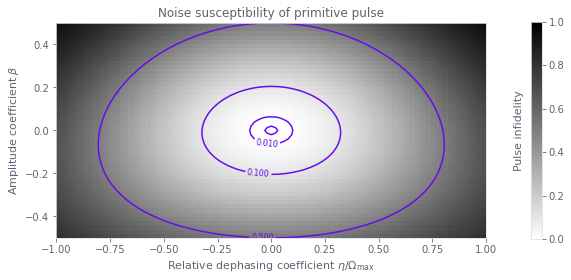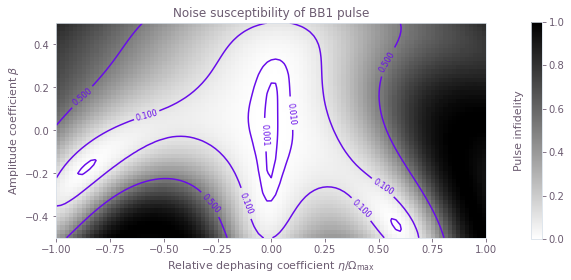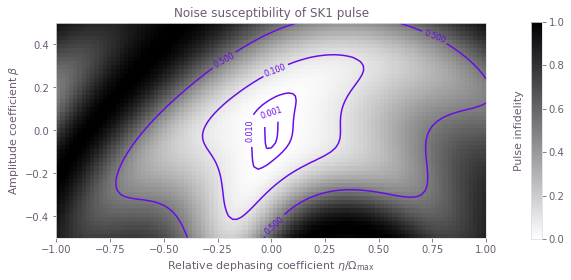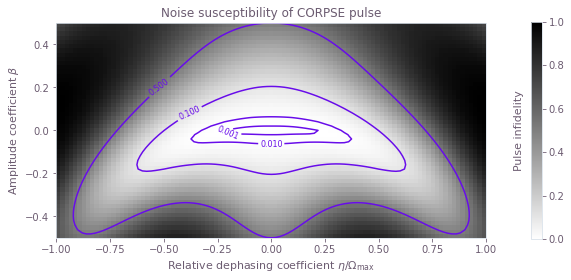### Summary

The plots demonstrate clearly the different noise susceptibilities of the different controls. For example, the BB1 control exhibits excellent robustness to amplitude noise (it retains a low infidelity across a wide range of amplitude noise values), but low dephasing robustness (infidelity increases rapidly as the dephasing coefficient varies from zero). The CORPSE control is the opposite: it is robust to dephasing noise, but highly susceptible to amplitude noise.

We have thus demonstrated how the Q-CTRL Python package can be used to characterize the robustness of different controls to quasi-static noise on multiple simultaneous noise channels.

## Example: Susceptibility to dephasing noise

The Q-CTRL Python package may also be used to perform quasi-static scans across a single noise channel. In this example we consider the simple case of a $\pi/2$ pulse performed on a single driven qubit experiencing dephasing noise. The system is described by the Hamiltonian:

\begin{align*} H(t) = & \frac{\Omega(t)}{2} \sigma_- + \frac{\Omega^*(t)}{2} \sigma_+ + \frac{\eta(t)}{2} \sigma_z \end{align*}

where $\Omega(t)$ is a time-dependent Rabi rate, $\eta(t)$ is a small, slowly-varying stochastic dephasing noise process, $\sigma_\pm = (\sigma_x \mp i \sigma_y)/2$, and $\sigma_k$ are the Pauli matrices.

In this case we consider only two driven controls, primitive and CORPSE.

## Define standard matrices
identity = np.array([[1, 0], [0, 1]], dtype=complex)
sigma_z = np.array([[1, 0], [0, -1]], dtype=complex)
sigma_m = np.array([[0, 1], [0, 0]], dtype=complex)
sqrt_sigma_x = 0.5 * np.array([[1 + 1j, 1 - 1j], [1 - 1j, 1 + 1j]], dtype=complex)

# Define control parameters
omega_max = 2 * np.pi * 1e6  # Hz
total_rotation = np.pi / 2

# Define coefficient array for the noise values
dephasing_coefficients = np.linspace(-1.0, 1.0, 101) * omega_max

# For each scheme, compute and plot the results of the quasi-static scan
for scheme_name, function in [
("CORPSE", qctrlopencontrols.new_corpse_control),
("primitive", qctrlopencontrols.new_primitive_control),
]:

# Define pulse objects using pulses from Q-CTRL Open Controls
pulse = function(
rabi_rotation=total_rotation,
azimuthal_angle=0.0,
maximum_rabi_rate=omega_max,
)

with qctrl.create_graph() as graph:

# Define Rabi coupling term
rabi_signal = qctrl.operations.pwc(
durations=pulse.durations,
values=pulse.rabi_rates * np.exp(1j * pulse.azimuthal_angles),
)
rabi_coupling_term = qctrl.operations.pwc_operator_hermitian_part(
rabi_signal * sigma_m
)

# Define dephasing term, a  batch of operators, created by multiplying
# a  batch of (constant) PWC signals by the corresponding σz/2 operator
dephasing_signal = qctrl.operations.constant_pwc(
constant=dephasing_coefficients,
duration=pulse.duration,
batch_dimension_count=1,
)
dephasing_term = dephasing_signal * sigma_z / 2

# Build total Hamiltonian, a  batch of operators
hamiltonian = rabi_coupling_term + dephasing_term

# Calculate infidelities, a  tensor
qctrl.operations.infidelity_pwc(
hamiltonian=hamiltonian,
target_operator=qctrl.operations.target(sqrt_sigma_x),
name="infidelities",
)

# Execute the graph
result = qctrl.functions.calculate_graph(
graph=graph, output_node_names=["infidelities"]
)

# Extract and plot infidelities
infidelities = result.output["infidelities"]["value"]
plt.plot(dephasing_coefficients / omega_max, infidelities, label=scheme_name)

plt.title("Dephasing noise susceptibility of different pulses")
plt.ylabel("Infidelity")
plt.xlabel(r"Relative dephasing coefficient $\eta/\Omega_\mathrm{max}$")
plt.legend()
plt.show()

Your task calculate_graph has completed.


Your task calculate_graph has completed.